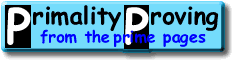Finding primes & proving primality 2.2: Fermat, probable-primality and pseudoprimesHome > Primality Proving > Chapter Two > Probable Primes

Fermat's "biggest", and also his "last" theorem states that xn + yn = zn has no solutions in positive integers x, y, z with n > 2.  This has finally been proven by Wiles in 1995 [Wiles95].  What concerns us here is his "little" theorem:

Fermat's (Little) Theorem:  If p is a prime and if a is any integer, then ap ≡ a (mod p).  In particular, if p does not divide a, then ap-1 ≡ 1 (mod p). ([proof])

Fermat's theorem gives us a powerful test for compositeness: Given n > 1, choose a > 1 and calculate an-1 modulo n (there is a very easy way to do quickly by repeated squaring, see the glossary page "binary exponentiation").  If the result is not one modulo n, then n is composite.  If it is one modulo n, then n might be prime so n is called a weak probable prime base a (or just an a-PRP).  Some early articles call all numbers satisfying this test pseudoprimes, but now the term pseudoprime is properly reserved for composite probable-primes.

The smallest examples of pseudoprimes (composite PRPs) are the following.  (There are more examples on the glossary page "probable prime ".)

• 341 = 11.31 is a 2-PRP, (Sarrus 1819)
• 91 = 7.13 is a 3-PRP,
• 217 = 7.31 is a 5-PRP and,
• 25 = 5.5 is a 7-PRP.

There are 1,091,987,405 primes less than 25,000,000,000; but only 21,853 pseudoprimes base two [PSW80], so Henri Cohen joked that 2-PRP's are "industrial grade primes" [Pomerance84, p5].  Fortunately, the larger n, the more likely (on the average) that a PRP test is correct--see the page "How probable?".

It is interesting to note that in 1950 Lehmer, using the weaker definition ana (mod n) for probable/pseudo-prime, discovered 2*73*1103 = 161038 is an even "pseudoprime" base two.  See [Ribenboim95 Chpt. 2viii] for a summary of results and history--including a debunking of the Chinese connection.  Richard Pinch listed the pseudoprimes to 1021 (by various definitions) at his website.

There may be relatively few pseudoprimes, but there are still infinitely many of them for every base a>1, so we need a tougher test.  One way to make this test more accurate is to use multiple bases (check base 2, then 3, then 5,...).  But still we run into an interesting obstacle called the Carmichael numbers.

Definition:  The composite integer n is a Carmichael number if an-1≡1 (mod n) for every integer a relatively prime to n.

Here is the bad news: repeated PRP tests of a Carmichael number will fail to show that it is composite until we run across one of its factors.  Though Carmichael number are 'rare' (only 2,163 are less than 25,000,000,000), it has recently been shown that there are infinitely many [AGP94].  The Carmichael numbers under 100,000 are

561, 1105, 1729, 2465, 2821, 6601, 8911, 10585, 15841, 29341, 41041, 46657, 52633, 62745, 63973, and 75361.

Richard Pinch listed the Carmichael's to 1016 (see [Pinch93]).

Note: Jon Grantham developed the idea of Frobenius Pseudoprime [Grantham2000] to generalize many of the standard types (Fermat, Lucas...), and to make the tests more accurate. His papers are available on-line.

 [ next page | previous page ] Copyright © 2023 PrimePages (Originally written by Chris Caldwell)